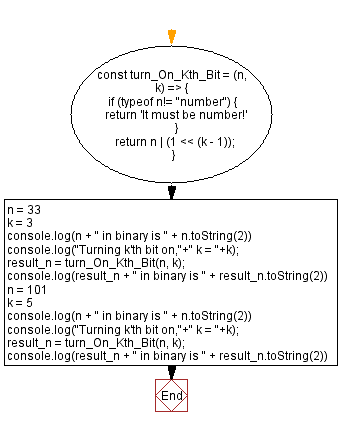# JavaScript - Turn on the kth bit of a given number

## JavaScript Bit Manipulation: Exercise-10 with Solution

Write a JavaScript program to turn on the kth bit of a given number. Return the updated number.

Test Data:
(33, 3)-> 37

Explanation:
Binary format of 33 is -> 100001
Fortmat of the said number after on the 3rd bit -> 100101
After converting 100101 to decimal : 100101 -> 37
(101, 5) -> 117
Explanation:
Binary format of 100 is -> 1100101
Fortmat of the said number after on the 5th bit -> 1110101
After converting 1110101 to decimal : 1110101 -> 117

Sample Solution:

JavaScript Code:

``````const turn_On_Kth_Bit = (n, k) => {
if (typeof n!= "number") {
return 'It must be number!'
}
return n | (1 << (k - 1));
}
n = 33
k = 3
console.log(n + " in binary is " + n.toString(2))
console.log("Turning k'th bit on,"+" k = "+k);
result_n = turn_On_Kth_Bit(n, k);
console.log(result_n + " in binary is " + result_n.toString(2))

n = 101
k = 5
console.log(n + " in binary is " + n.toString(2))
console.log("Turning k'th bit on,"+" k = "+k);
result_n = turn_On_Kth_Bit(n, k);
console.log(result_n + " in binary is " + result_n.toString(2))
```
```

Sample Output:

```33 in binary is 100001
Turning k'th bit on, k = 3
37 in binary is 100101
101 in binary is 1100101
Turning k'th bit on, k = 5
117 in binary is 1110101
```

Flowchart:Live Demo:

See the Pen javascript-bit-manipulation-exercise-10 by w3resource (@w3resource) on CodePen.

* To run the code mouse over on Result panel and click on 'RERUN' button.*

Improve this sample solution and post your code through Disqus

What is the difficulty level of this exercise?

Test your Programming skills with w3resource's quiz.

﻿